# High School Math : Basic Statistics

## Example Questions

### Example Question #1 : Using Scatter Plots

Based on the scatter plot below, is there a correlation between theandvariables? If so, describe the correlation.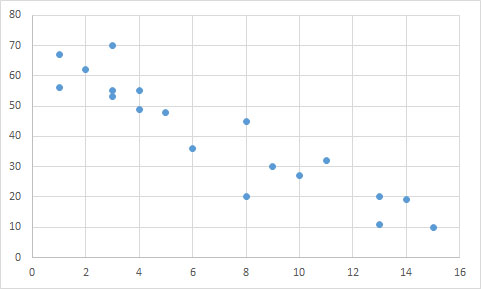Yes; negative linear relationship

Yes; positive linear relationship

Yes; negative exponential relationship

No; there is no correlation

Yes; negative linear relationship

Explanation:

The data points follow an overall linear trend, as opposed to being randomly distributed. Though there are a few outliers, there is a general relationship between the two variables.

A line could accurately predict the trend of the data points, suggesting there is a linear correlation. Since the y-values decrease as the x-values increase, the correlation must be negative. We can see that a line connecting the upper-most and lower-most points would have a negative slope.

An exponential relationship would be curved, rather than straight.

### Example Question #1 : How To Find The Range For A Set Of Data

Six homes are for sale and have the following dollar values in thousands of dollars:

535

155

305

720

315

214

What is the range of the values of the six homes?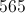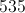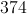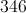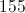Explanation:

The range is the simplest measurement of the difference between values in a data set.  To find the range, one simply subtracts the lowest value from the greatest value, ignoring the others.  Here, the lowest value is 155 and the greatest is 720.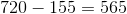### Example Question #2 : How To Find The Range For A Set Of Data

Alice recorded the outside temperature at noon each day for one week. These were the results.

Monday: 78

Tuesday: 85

Wednesday: 82

Thursday: 84

Friday: 82

Saturday: 79

Sunday: 80

What is the range of temperatures?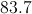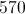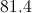Explanation:

The range is the simplest measurement of the difference between values in a data set.  To find the range, simply subtract the lowest value from the greatest value, ignoring the others.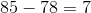### Example Question #1 : Understanding Range

You are given the following data: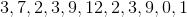What is the range?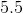Explanation:

Recall that we find the range of a set of data by subtracting the smallest value from the largest value. In this case, the smallest value isand the largest value is. Thus, our range is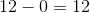.

### Example Question #2 : Understanding Range

There aregirls andboys running a race.  What is the probability that only boys will finish in the top three places?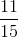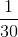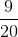Explanation:

Probability is a fraction betweenand.  The numerator is the total number of ways to get what you want, or a subset of the sample space.  The denominator is the total ways, or complete sample space.

The number of ways only boys would finsih in the top three places: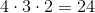The number of ways all contestants can finish the race: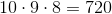So the probability of only boys finishing in the top three places is given by the fraction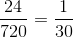when simplified.

### Example Question #1 : Understanding Mean, Median, And Modeis the ____________ of the following dataset below.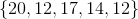range

mode

mean

median

median

Explanation:

Reorder the numerals in the set, from least to greatest.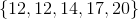The number in the middle is the median.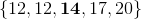The most frequent numeral is the mode.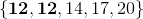The mean is the sum of the numerals divided by the number of data points.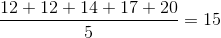The mean is 15.

The range is the difference between the maximum and the minimum.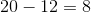The range is 8.

### Example Question #2 : Understanding Mean, Median, And Mode

For the following data set: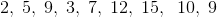Which is the smallest?

Median

Mode

Range

Mean

Mean

Explanation:

Put the data in order from smallest to largest and then calculate each stastic:  mean, mode, median, range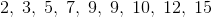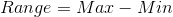or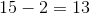Mode is the most often repeated number orMedian is the number in the middle orMean is the sum of the data divided by the number of data points or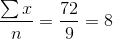### Example Question #3 : Understanding Mean, Median, And Mode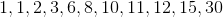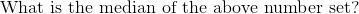Explanation: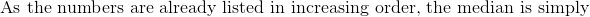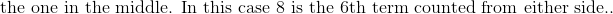### Example Question #4 : Understanding Mean, Median, And Mode

Find the median of the following number series:

3, 6, 27, 19, 8, 11, 30, 42, 7, 39

11

19

15

30

19.2

15

Explanation:

The first step to finding the median is always to put the numbers in the proper order:

3, 6, 7, 8, 11, 19, 27, 30, 39, 42

When we have an even amount of numbers, we find the average (or mean) of the middle two to get the median:

11 + 19 = 30/2 = 15

### Example Question #5 : Understanding Mean, Median, And Mode

With a standard deck of playing cards, what is the probability of picking one red card followed by one black card, without replacement?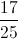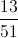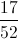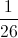Explanation:

In a standard deck of playing cards we have: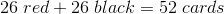So the probability of picking the first card red is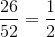.

Then the probability of picking the second card black is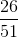because this is without replacement.

They are independent events so the probabilities get multiplied together to give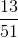.